RS Aggarwal Class 6 Solutions Chapter 12 - Parallel Lines

RS Aggarwal Class 6 Chapter 12 - Parallel Lines Solutions Free PDF

Parallel lines is one of the most important concepts of mathematics. Learning the properties of parallel lines will help the students to understand the concepts of geometry in an easy and better way. To practice questions based on parallel lines, students are advised to solve questions from RS Aggarwal for class 6. To help students understand all the concepts and to solve all the questions we have also provided the RS Aggarwal Class 6 solutions chapter 12 parallel lines.

EXERCISE 12

Question 1:

In the figure of a table given below, name the pairs of parallel edges of the top.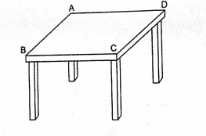Solution:

Following are the parallel edges of the top:

This is because AD and BC will not intersect even if both these line segments are produced to infinity in both the directions.

AB||DC

This is because AB and DC will not intersect even if both these line segments are produced to infinity in both the directions.

Question 2:

Name the groups of all possible parallel edges of the box whose figure is shown below.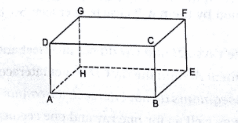Solution:

The groups of parallel edges are (AD||GH||BC||FE), (AB||DC||GF||HE) and (AH||BE||CF||DG).

The above mentioned groups of edges are parallel because they will not meet each other if produced to infinity to both sides.

Question 3:

Identify parallel line segments in each of the figures given below: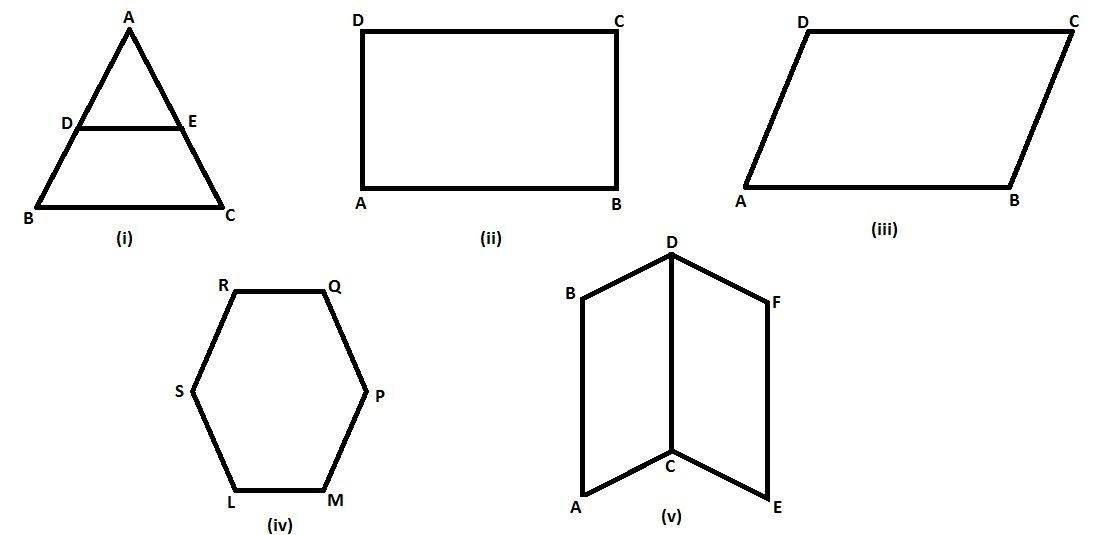Solution:

(i)

DE||BC

This is because they do not intersect each other.

(ii)

This is because these pairs of line segments do not intersect each other.

(iii)

This is because these pairs of line segments do not intersect each other.

AB does not intersect DC and AD does not intersect BC.

(iv)

LM||RQ, RS||PM and LS||PQ

These pairs of line segments are non-intersecting.

So, these pairs of lines are parallel.

(v)

AB||DC, AB||EF, DC||EF

AC||BD, CE||DF

These pairs of line segments are non-intersecting.

So, these pairs of lines are parallel.

Question 4:

Find the distance between the parallel lines l and m, using a set square.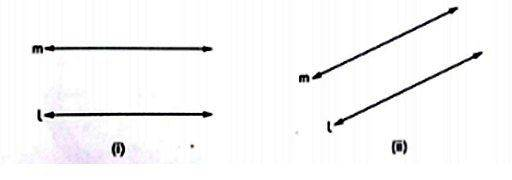Solution:

(i) Distance between l and m is 1.3 cm.

Place the ruler so that one of its measuring edges lies along the line l. Hold it with one hand. Now place a set square with one arm of the right angle coinciding with the edge of the ruler. Draw the line segment PM along the edge of the set square, as shown in the figure. Then, measure the distance (PM) between l and m, which will be equal to 1.3 cm.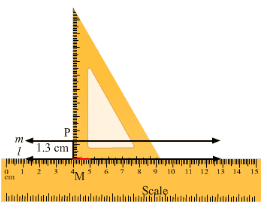(ii) Distance between l and m is 1 cm.

Place the ruler so that one of its measuring edges lies along the line l. Hold it with one hand. Now place a set square with one arm of the right angle coinciding with the edge of the ruler. Draw the line segment PM along the edge of the set square, as shown in figure. Then, measure the distance (PQ) between l and m as 1 cm.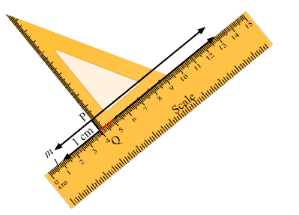Question 5:

In the figure, l || m. If AB $perp$ l, CD $perp$ l and AB = 2.3 cm, find CD.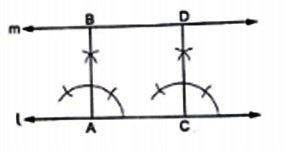Solution:

At point A, AB is the perpendicular distance between l and m.

At point C, CD is the perpendicular distance between l and m.

The perpendicular distance between two parallel lines is same at all points.

Therefore, CD = AB = 2.3 cm

Question 6:

In the figure, do the segments AB and CD intersect? Are they parallel? Give reason for your answer.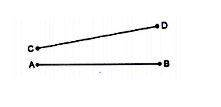Solution:

Line segments AB and CD will intersect if they are produced endlessly towards the ends A and C, respectively.

Therefore, they are not parallel to each other.

Question 7:

Using a set square and a ruler, test whether l || m in each of the following cases: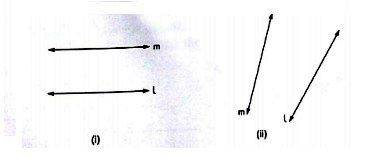Solution:

(i) Place the ruler so that one of its measuring edges lies along the line l. Hold it firmly with one hand. Now place a set square with one arm of the right angle coinciding with the edge of the ruler. Draw line segments between l and m (say PM, RS, AB) with the set square.

Now, we see that PM = AB = RS.

Thus, we can say that l || m.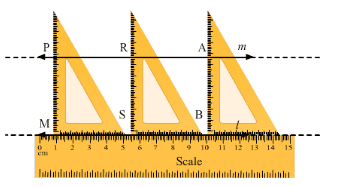(ii) In this case, we see that when we draw line segments between l and m, they are unequal, i.e., PM $neq$ RS.

Therefore, l is not parallel to m.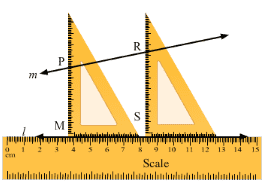Question 8:

Which of the following statements are true and which are false?

(i) Two lines are parallel if they do not meet, even when produced.

(ii) Two parallel lines are everywhere the same distance apart.

(iii) If two line segments do not intersect, they are parallel.

(iv) If two rays do not intersect, they are parallel.

Solution:

(i) True

The statement is true because such lines do not intersect even when produced.

(ii) True

Perpendicular distance between two parallel lines is same at all points on the lines.

(iii) True

If the corresponding lines are produced infinitely, they will not intersect. Hence, they are parallel.

(iv) True

The corresponding lines determined by them will not intersect. Hence, they are parallel to each other.’

Practise This Question

The poster below gives out a message on: